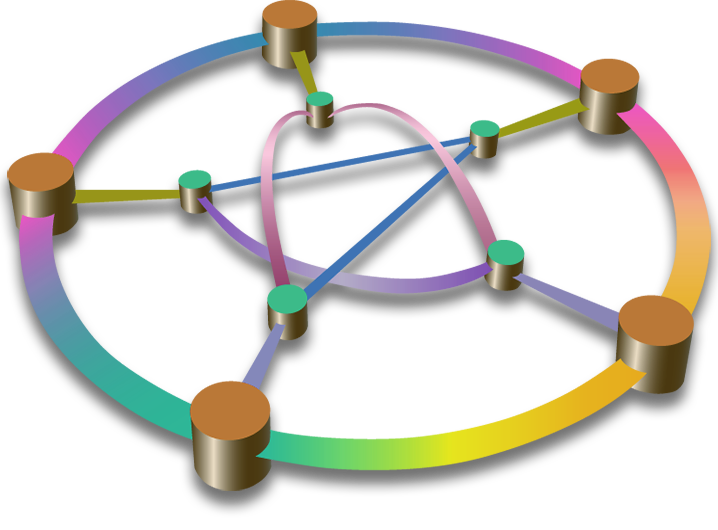Mathematics
Te Tari Pāngarau me te Tatauranga
Department of Mathematics & Statistics

## Which MATH and COMO 100-level papers should I take?

The following 100-level Math papers are offered every year (in increasing order of level of Mathematics):

Note: MATH 130 must be completed and passed before enrolling in MATH 140. MATH 120 is not a pre-requisite for MATH 130, but it can be an excellent bridging paper for students lacking some background or confidence in mathematics.

MATH 120 uses a problem-based learning approach to develop problem-solving and critical thinking skills by consistently working through applied examples from a range of scientific disciplines, while learning new techniques and tools. This paper is ideal for students wanting to build confidence in mathematics. It demonstrates how mathematical skills and ideas are relevant to a huge range of contexts.

MATH 130 and MATH 140 cover the fundamental mathematical concepts and skills which are standard nationally and internationally. Level 2 NCEA Mathematics or equivalent is expected, although level 3 mathematics would be preferred. Internationally, this material would be expected background of essentially any science, engineering or commerce students. One or both papers are required for numerous degree programs at Otago. The paper covers key concepts in algebra, geometry, logic and calculus, building the foundations for applied and theoretical work both within science and beyond.

COMO 101 teaches mathematical modelling and simulation. Modelling skills are currently in high demand, we've seen their importance in strategies for dealing with pandemics and climate change. However pretty much any application of mathematical tools to real-life problems requires some aspect of modelling.

Whatever your circumstances are, if you are still unsure, please feel free to talk to one of our Mathematics advisers.